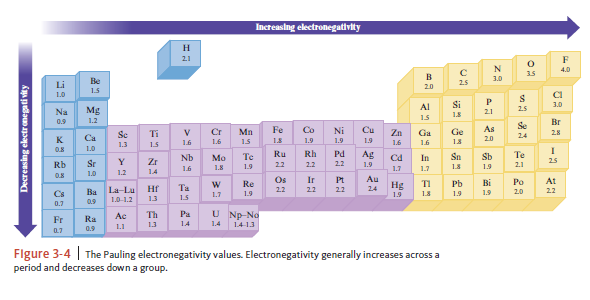# Problem: Exercise 31. Without using Fig. 3‑4, predict the order of increasing electronegativity in each of the following groups of elements.a. C, N, Ob. S, Se, Clc. Si, Ge, Snd. Tl, S, GeExercise 33. Without using Fig. 3‑4, predict which bond in each of the following groups will be the most polar.a. C—F, Si—F, Ge—Fb. P—Cl or S—Clc. S—F, S—Cl, S—Brd. Ti—Cl, Si—Cl, Ge—ClRepeat Exercises 31 and 33, this time using the values for the electronegativities of the elements given in figure below. Are there differences in your answers?

98% (59 ratings)
###### Problem Details

Exercise 31. Without using Fig. 3‑4, predict the order of increasing electronegativity in each of the following groups of elements.

a. C, N, O

b. S, Se, Cl

c. Si, Ge, Sn

d. Tl, S, Ge

Exercise 33. Without using Fig. 3‑4, predict which bond in each of the following groups will be the most polar.

a. C—F, Si—F, Ge—F

b. P—Cl or S—Cl

c. S—F, S—Cl, S—Br

d. Ti—Cl, Si—Cl, Ge—Cl

Repeat Exercises 31 and 33, this time using the values for the electronegativities of the elements given in figure below. Are there differences in your answers?